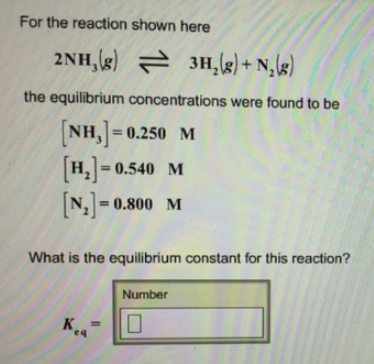# Problem: For the reaction shown here 2NH3 (g) ⇌ 3 H2 (g) + N2 (g) the equilibrium concentrations were found to be [NH3] = 0.250 M [H2] = 0.540 M [N2] = 0.800 M What is the equilibrium constant for this reaction?

###### FREE Expert Solution###### Problem Details

For the reaction shown here

2NH3 (g) ⇌ 3 H2 (g) + N2 (g)

the equilibrium concentrations were found to be

[NH3] = 0.250 M

[H2] = 0.540 M

[N2] = 0.800 M

What is the equilibrium constant for this reaction?